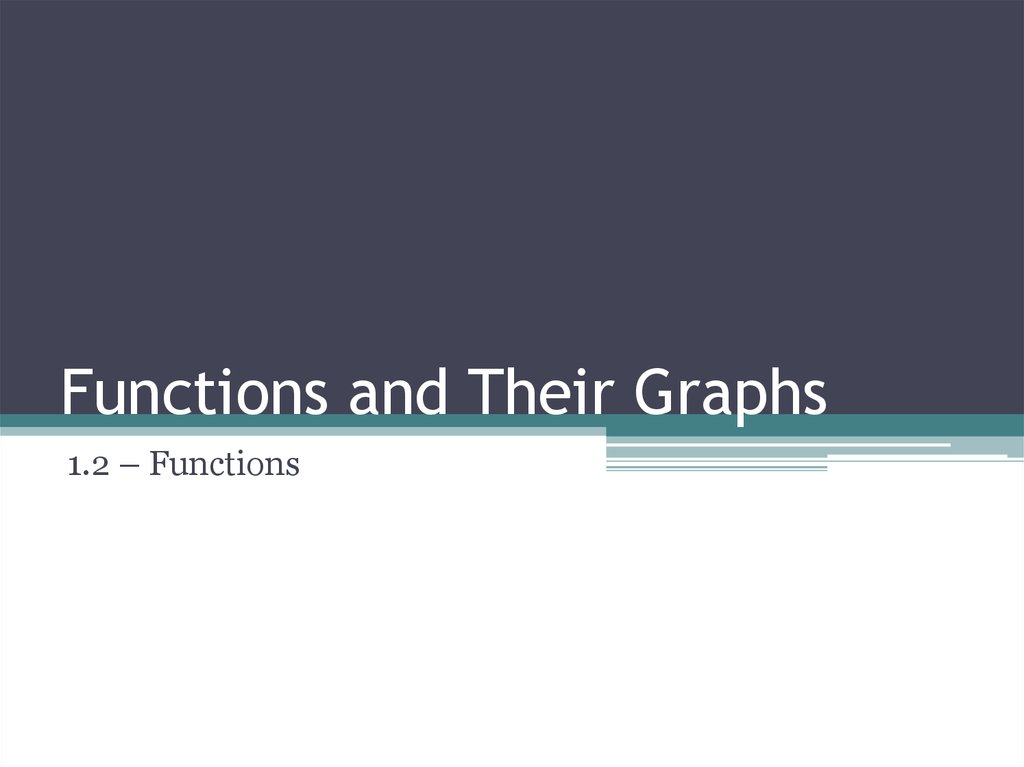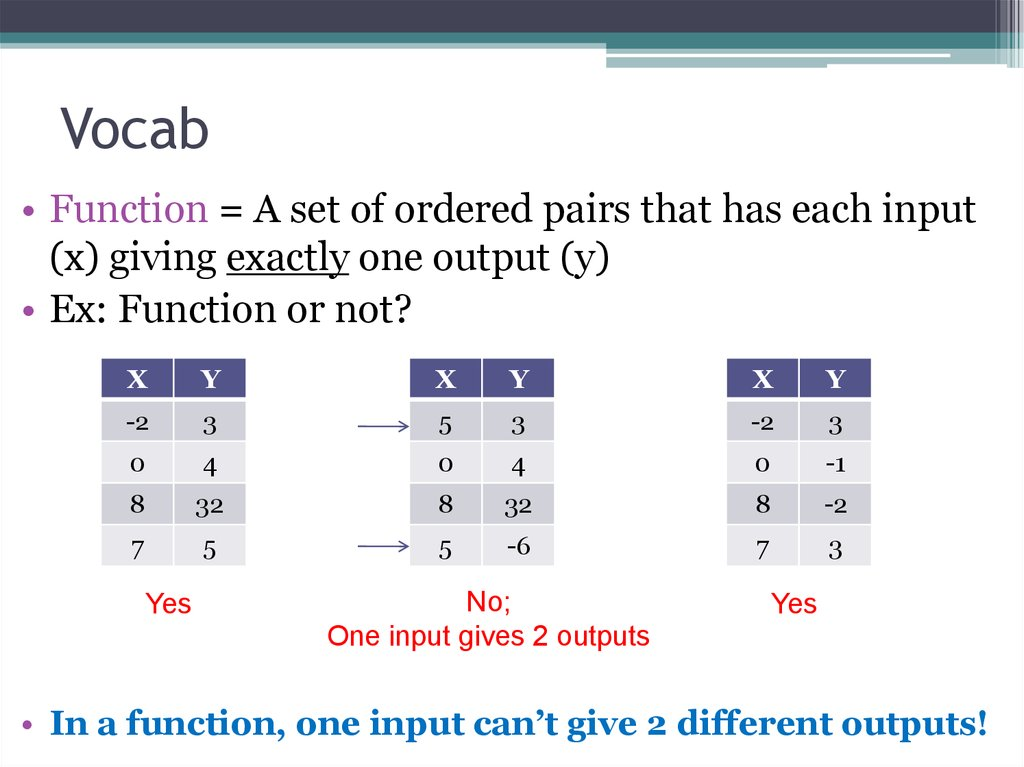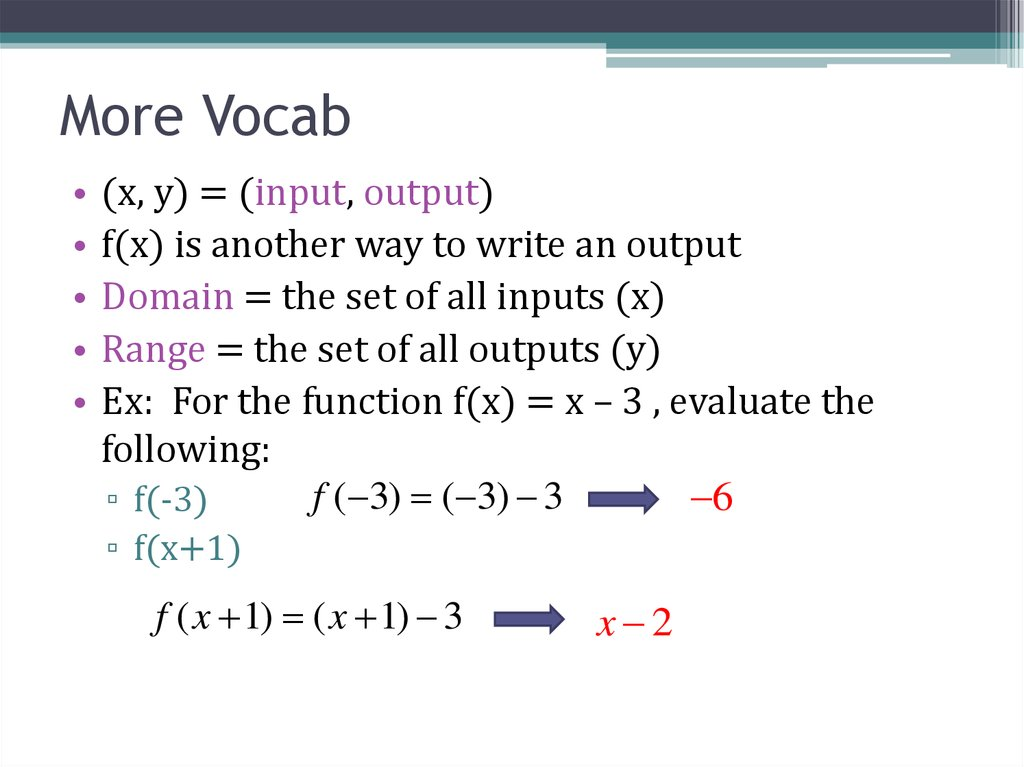# Functions and their graphs

1.2 – Functions

## 2. Vocab

• Function = A set of ordered pairs that has each input
(x) giving exactly one output (y)
• Ex: Function or not?
X
Y
X
Y
X
Y
-2
3
5
3
-2
3
0
4
0
4
0
-1
8
32
8
32
8
-2
7
5
5
-6
7
3
Yes
No;
One input gives 2 outputs
Yes
• In a function, one input can’t give 2 different outputs!

## 3. More Vocab

(x, y) = (input, output)
f(x) is another way to write an output
Domain = the set of all inputs (x)
Range = the set of all outputs (y)
Ex: For the function f(x) = x – 3 , evaluate the
following:
f ( 3) ( 3) 3
6
▫ f(-3)
▫ f(x+1)
f ( x 1) ( x 1) 3
x 2

## 4.

• Ex: For the function f(x) = 2 – x2 , evaluate the
following:
▫ f(x+1)
f ( x 1) 2 ( x 1)2
2 ( x 2 2 x 1)
2 x 2x 1
2
x2 2x 1
• Ex: For the function f(x) = x2 + x , evaluate the
following:
▫ f(2x)
f (2 x) (2 x) (2 x)
2
4x2 2x

## 5.

• Ex: For the function f(x) = x2 – 2x + 3, evaluate
the following:
▫ f(x+h)
f ( x h) ( x h) 2( x h) 3
2
x 2 xh h 2 x 2h 3
2
2

## 6.

• Ex: For the function f(x) = 2x2 – 3 , evaluate the
following:
▫ The difference quotient f ( x h) f ( x)
h
(2( x h) 2 3) (2 x 2 3)
h
(2( x 2 2hx h 2 ) 3) 2 x 2 3
h
2 x 2 4hx h 2 3 2 x 2 3
h
4hx h 2
h
4x h

5x – 3
5x + 3
5x – 9
5x – 15
15
0%
5x

9
0%
5x

3
0%
5x
+
3
0%
5x

1.
2.
3.
4.

0%
0%
-x
2
+4
x+
-x
2
+2
x+
1
0%
-x
2
+1
0%
3
-x2 + 1
-x2 + 2x + 1
-x2 +4x + 3
-x2
-x
2
1.
2.
3.
4.

## 9.

• Ex: The function below is a piecewise function.
Find f(0) and f(1).
x 3, x 1
f ( x)
2 x 4, x 1
▫ Since 0<1, use the top function for f(0).
▫ f(0) = -3!
▫ Since 1≥1, use the bottom function for f(1).
▫ f(1) = -2!

## 10. More Vocab

• y = x2 means y is a function of x
• Y is not a function of x when a ± is in play
• Ex: Which of these has y as a function of x?
▫ x2 – y = 7
Solve for y first…
- y = 7 – x2
y = x2 – 7 … no ± means YES!
▫ x2 + y2 = 2x
y2 = 2x – x2
y = 2 x x 2 … so NO!

## 11. Finding Domain and Range

• The domain (set of all x’s) is always assumed to be all
real numbers unless some values cannot create
outputs (y’s).
• Ex: Find the domain of the following functions:
▫ y = 2x – 3
Any x will produce a y, so the domain is xϵℝ (all reals)
▫ y=
x
The square root can’t be negative, so the domain is x≥0
▫ y=
3
2x 4
The denominator can’t be 0, so 2x – 4 ≠0…
…x≠2

## 12. Finding Domain and Range

• To find range, graph the function and infer the range
(set of all y’s).
• Ex: Find the domain and range of the function y x 3
▫ Graph the function first.
▫ For the domain, we know from the equation given that
x ≥ 3. Our graph confirms that.
▫ For the range, the graph shows us
that there are no negative values
for y, and the values will continue
to increase as x increases.
▫ Range: y ≥ 0

## 13. What is the domain?

f ( x) 4 x
2
What is the domain?
1.
2.
3.
4.
xϵℝ
-2≤x≤2
x≥0
-2<x<2

## 14. What is the domain?

x 2
f ( x)
x 3
What is the domain?
1.
2.
3.
4.
xϵℝ
x ≠ -2
x≠3
x ≠ -2 and x ≠ 3

## 15. What is the range?

f ( x) 2 x 5
2
What is the range?
1.
2.
3.
4.
yϵℝ
y≠5
y < -5
y ≥ -5

## 16. Ch. 1 – Functions and Their Graphs

1.3 – More Functions

## 17. Vertical Line Test

• Vertical is up and down!
• Vertical Line Test: If you can draw some vertical line on
a graph and it goes through MORE THAN ONE point,
the graph is NOT a function.
▫ Ex: Are these graphs functions?
YES!
YES!
NO!

## 18. Vocab

• As we read left to right, the function
to the right is…
▫ …decreasing in the red region
Decreasing for x<-1, so we write ( , 1) to
indicate that y decreases over that x interval
▫ …constant in the blue region
Constant for -1≤x≤2, so we write
( 1, 2)
▫ …increasing in the green region
Increasing for x>2, so we write
(2, )

## 19. Vocab

• When a function goes from increasing to
decreasing (or visa versa), it will have a relative
minimum or a relative maximum.
• The graph below has a relative maximum at (-2, 2)
and a relative minimum at (1, -2).
• A graph can have any amount of
relative minima or maxima.

## 20. Functions

• A function is even if it is
▫ f(-x) = f(x)
• A function is odd if it is
▫ f(-x) = -f(x)
• A graph symmetric about the xaxis is…
▫ …not a function!

## 21. The function y = 4x2 – 2 is…

Even
Odd
None of the above
Not a function
fu
nc
t io
a
No
t
bo
ve
ea
th
of
0%
n
0%
Od
d
0%
No
ne
n
0%
Ev
e
1.
2.
3.
4.

## 22. The function y = 1/x is…

Even
Odd
None of the above
Not a function
fu
nc
t io
a
No
t
bo
ve
ea
th
of
0%
n
0%
Od
d
0%
No
ne
n
0%
Ev
e
1.
2.
3.
4.

## 23. The function y = x3 – x is…

Even
Odd
None of the above
Not a function
fu
nc
t io
a
bo
ve
ea
th
0%
n
0%
No
t
0%
of
Ev
e
n
0%
Od
d
Figure it out algebraically –
no graphing!!!
No
ne
1.
2.
3.
4.